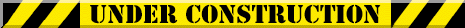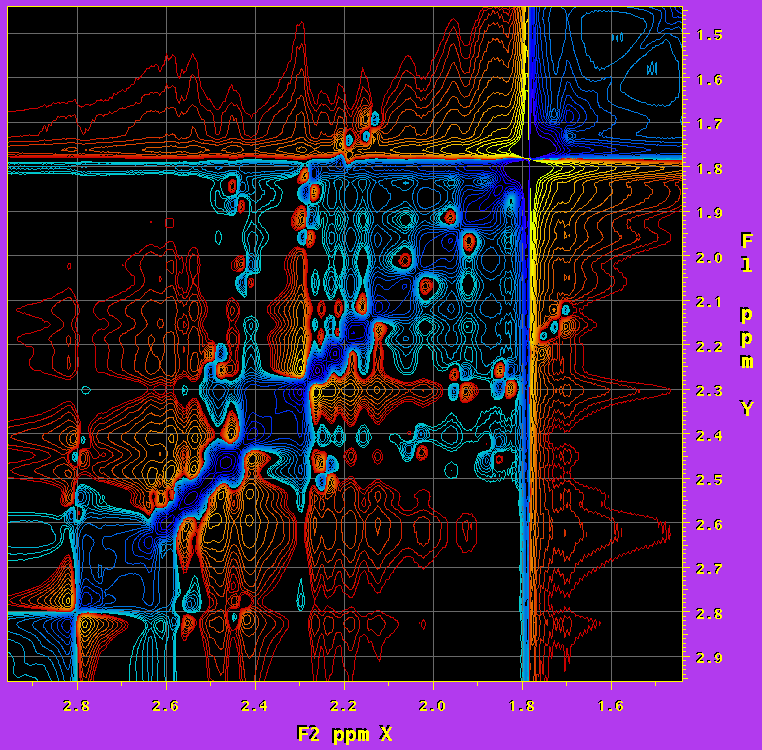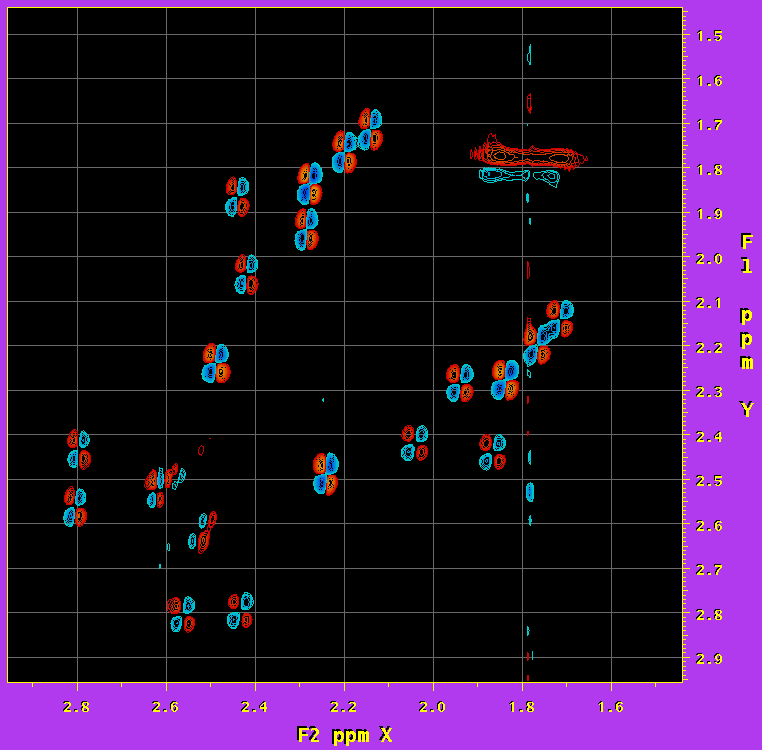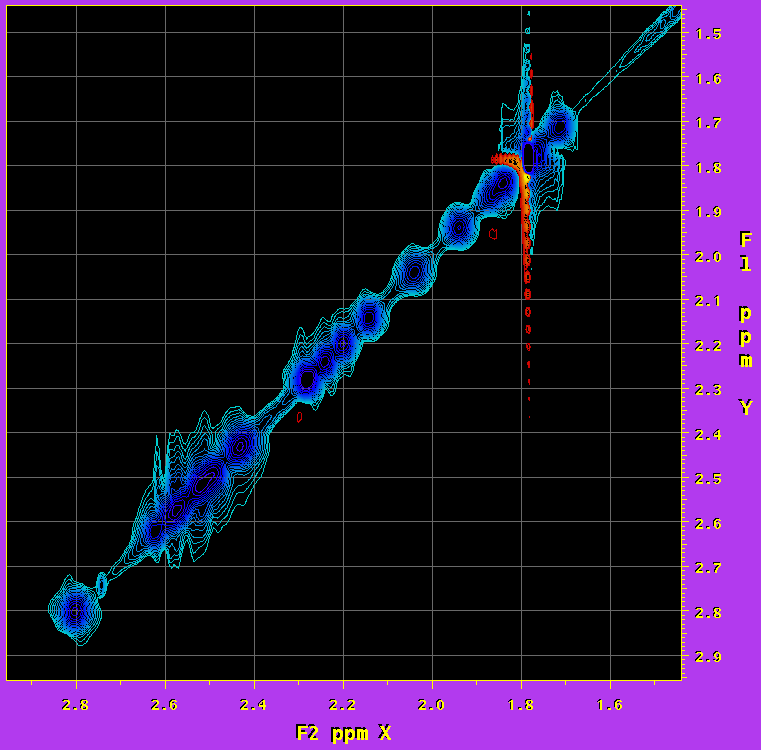# Numerical Diagonal Suppression in COSY

Under the gentle guidance and encouragement of Ad Bax, we've been revisiting the problem of measuring proton-proton couplings from traditional COSY spectra. Of course, the problem in COSY spectra is well known: In both frequency dimensions, the diagonal is 90s out of phase relative to the cross peaks. So, when phasing the cross peaks to be absorptive, the tails from the dispersive diagonal peaks obscure many of the nearby cross peaks (see top panel of Figure). A long, long time ago, Luciano Mueller suggested an elegant way of subtracting the diagonal by conducting a COSY experiment without a mixing pulse (1). Alternatively, such a no-mixing pulse "COSY" spectrum can be generated from a single FID (2). Unfortunately, in Ad's soupy samples, which besides our little molecules contain liquid crystal such as bicelles or phages, this trick does not work so well. Instead, we therefore went back and tried to attenuate the diagonal signals numerically. We've had good results using a scheme that combines frequency shifting with methods usually used for solvent signal subtraction, such as the time-domain convolution method (3) and time-domain polynomial subtraction (4). As you know, these solvent subtraction methods are designed to suppress low-frequency signals, i.e. those at the center of the spectrum. Therefore, if we temporarily shift columns of a 2D spectrum in such a way that the diagonal signal is moved to the center, these low-frequency suppression methods can also be used for diagonal suppression. This means we need to apply an amount of shifting that varies according to the position of the diagonal signal in any given column (vector). Since frequency shifting can be achieved by a first-order phase correction in the time domain, the diagonal suppression steps can all be carried out in the t1 time domain. In practice, after the t2 Fourier transformation and absorptive phasing, the frequency shifting, diagonal subtraction, and frequency unshifting are applied to the t1 vectors of the 2D data matrix.

We've implemented this scheme using facilities of our software system NMRPipe. This may be especially apropos, since interestingly (if you find obscure NMR software history interesting) the very first application of our pipeline-based software was implementation of a diagonal zeroing method by Mitsu Ikura and Istvan Pelczer, a close relative of the current scheme. In any event, a complete description of NMRPipe in its current form, including details on how to decipher processing schemes such as the ones here, can be found at: http://spin.niddk.nih.gov/bax/software.

An example UNIX processing pipeline script for our 2D COSY processing is shown below. In the scheme, the directly-detected dimension is processed as usual, but with the signals of the first t1 increment phased absorptively after the first FT. Then, each t1 vector in the indirect dimension is shifted via phase correction so that its diagonal signal becomes on-resonance, a "solvent" filter is applied by subtracting a best-fit 4th or 5th order polynomial, and the vector is shifted back again. The solvent filter methods sometimes distort intensities at the head and tail of the time-domain data, leading to baseline curvature near the region of signal suppression in the spectrum. So in this case, since the signals of interest are sine-modulated, the leading points of the time-domain data can be attenuated with a suitable window without affecting the cross peak signals. Here, we used a custom roll-off function with a cosine-squared form. After these diagonal suppression steps, the t1 vectors are then processed as usual, and finally, the direct dimension is rephased to dispersive mode. The script is annotated to describe the processing steps, with the functions applied to the indirect dimension given in bold:

nmrPipe -in test.fid                                \ Read FID
| nmrPipe -fn SP -off 0.5 -pow 2 -end 0.95 -c 0.5   \ Window, 1st Point Scale
| nmrPipe -fn ZF -size 2048                         \ Zero Fill
| nmrPipe -fn FT -verb                              \ Fourier Transform
| nmrPipe -fn PS -p0 138.7 -p1 18.1 -di             \ F2 Phase Correction
| nmrPipe -fn TP                                    \ 2D Transpose
| nmrPipe -fn MAC -macro diagShift.M                \ Shift Diagonal to Center
| nmrPipe -fn POLY -time                            \ Polynomial Solvent Filter
| nmrPipe -fn MAC -macro diagUnShift.M              \ Shift Diagonal Back
| nmrPipe -fn MAC -macro csr.M -var wide 10         \ Attenuate Head of FID
| nmrPipe -fn SP -off 0.5 -pow 2 -end 0.95 -c 1.0   \ Window
| nmrPipe -fn ZF -size 2048                         \ Zero Fill
| nmrPipe -fn FT -verb                              \ Fourier Transform
| nmrPipe -fn PS -p0 -110 -p1 220 -di               \ F1 Phase Correction
| nmrPipe -fn POLY -auto                            \ Auto Baseline Correction
| nmrPipe -fn TP                                    \ 2D Transpose
| nmrPipe -fn POLY -auto                            \ Auto Baseline Correction
| nmrPipe -fn PS -p0 90 -ht -di                     \ Rephase F2 to Dispersive
-out test.ft2 -verb -ov                            Write Spectrum

We decided to implement the shifting operations using the macro interpreter facility of NMRPipe, which allows custom functions to be included in processing schemes without the need to compile new versions of the software. The macro language is a subset of the C programming language, augmented with a number of vector processing functions. The shifting macro diagShift.M is shown below; it is invoked once for each t1 vector in the interferogram. The macro language provides many automatically defined variables that describe the current data. In this case, the variables xSize and ySize give the number of points in t1 and f2 respectively, and yLoc gives the index number of the t1 vector being processed, in the range of 1 to ySize. The variables rdata and idata are arrays containing the real and imaginary parts of the current vector. Finally, phase( ) is a vector processing function which applies a zero and first order correction as given in degrees:

xMid   = 1 + xSize/2;
yMid   = 1 + ySize/2;

slope  = (1 - xMid)/(yMid - 1);
offset = -slope*yMid;
shift  = slope*yLoc + offset;

p0     = 0.0;
p1     = -360.0*shift;

(void) phase( rdata, idata, xSize, p0, p1 );

The cosine-squared roll-off function was also implemented as a macro, csr.M, shown here. This function multiplies the data by a function that increases from zero to one over the first several points in the data, and leaves the remainder of the data unchanged. The number of points attenuated is specified by the variable wide, which is taken from the command-line of the processing script:

for( i = 0; i < wide; i++ )
{
c = cos( 0.5*PI*i/wide );
w = 1.0 - c*c;

rdata[i] *= w;
idata[i] *= w;
}

On the right, an example result is shown for a diagonal region in a 2D COSY of a DNA sample, spanning 2.96 to 1.44 ppm. The topmost contour plot shows the result of conventional processing, which predictably is dominated by the diagonal, making the crosspeaks very hard to see or measure. The central contour plot shows the same data, as processed with the diagonal suppression scheme. The crosspeaks here are easily seen, and in this case even those close to the diagonal can be quantified. The contour plot at bottom shows the diagonal signal alone, as generated by taking the difference between the original and diagonal suppressed data, then rephasing both dimensions to absorptive mode. In this case, the diagonal is well isolated and undistorted, and can also be quantified. All three plots have been drawn with the same contour parameters.(1) L. Mueller, J. Magn. Reson. 72, 191 (1987).

(2) D. Marion and A. Bax, J. Magn. Reson. 80, 528-533 (1988).

(3) Marion D., Ikura, M., and Bax, A., J. Magn. Reson. 84, 425-430 (1989).

(4) Callaghan, P.T., MacKay, A.L., Pauls, K.P., Soderman, O., and Bloom, M., J. Magn. Reson. 56, 101-109 (1984).